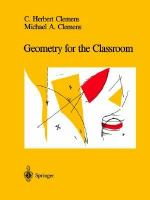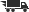Close

# Geometry for the Classroom

## by C. Herbert Clemens• ISBN: 9780387975641
• ISBN10: 0387975640

# Geometry for the Classroom

## by C. Herbert Clemens

• Binding: Paperback
• Publisher: Springer Verlag
• Publish date: 05/01/1991
• ISBN: 9780387975641
• ISBN10: 0387975640
used \$5.43
You save: 93%
new \$90.17
FREE economy shipping!
Description: Intuition.- I1: Geometry is about shapes.- I2:... and more shapes.- I3: Polygons in the plane.- I4: Angles in the plane.- I5: Walking north, east, south, and west in the plane.- I6: Areas of rectangles.- I7: What is the area of the shaded triangle'.- I8: Adding the angles of a triangle.- I9: Pythagorean theorem.- I10: Side Side Side (SSS).- I11: Parallel lines.- I12: Rectangles between parallels and the Z-principle.- I13: Areas: The principle of parallel slices.- I14: If two lines in the plane do not intersect, they are parallel.- I15: The first magnification principle: preliminary form.- I16: The first magnification principle: final form.- I17: Area inside a circle of radius one.- I18: When are triangles congruent'.- I19: Magnifications preserve parallelism and angles.- I20: The principle of similarity.- I21: Proportionality of segments cut by parallels.- I22: Finding the center of a triangle.- I23: Concurrence theorem for altitudes of a triangle.- I24: Inscribing angles in circles.- I25: Fun facts about circles, and limiting cases.- I26: Degrees and radians.- I27: Trigonometry.- I28: Tangent a =(rise)/(run).- I29: Everything you always wanted to know about trigonometry but were afraid to ask.- I30: The law of sines and the law of cosines.- I31: Figuring areas.- I32: The second magnification principle.- I33: Volume of a pyramid.- I34: Of cones and collars.- I35: Sphereworld.- I36: Segments and angles in sphereworld.- I37: Of boxes, cylinders, and spheres.- I38: If it takes one can of paint to paint a square one widget on a side, how many cans does it take to paint a sphere with radius r widgets'.- I39: Excess angle formula for spherical triangles.- I40: Hyperbolic-land.- Construction.- C1: Copying triangles.- C2: Copying angles.- C3: Constructing perpendiculars.- C4: Constructing parallels.- C5: Constructing numbers as lengths.- C6 Given a number, construct its square root.- C7: Constructing parallelograms.- C8: Constructing a regular 3-gon and 4-gon.- C9: Constructing a regular 5-gon.- C10: Constructing a regular 6-gon.- C11: Constructing a regular 7-gon (almost).- C12: Constructing a regular tetrahedron.- C13: Constructing a cube and an octohedron.- C14: Constructing a dodecahedron and an icosahedron.- C15: Constructing the baricenter of a triangle.- C16: Constructing the altitudes of a triangle.- C17: Constructing a circle through three points.- C18: Bisecting a given angle.- C19: Putting circles inside angles.- C20: Inscribing circles in polygons.- C21: Circumscribing circles about polygons.- C22: Drawing triangles on the sphere.- C23: Constructing hyperbolic lines.- Proof.- P1: Distance on the line, motions of the line.- P2: Distance in the plane.- P3: Motions of the plane.- P4: A list of motions of the line.- P5: A complete list of motions of the line.- P6: Motions of the plane: Translations.- P7: Motions of the plane: Rotations.- P8: Motions of the plane: Vertical flip.- P9: Motions of the plane fixing (0,0) and (a,0).- P10: A complete list of motions of the plane.- P11: Distance in space.- P12: Motions of space.- P13: The triangle inequality.- P14: Co-ordinate geometry is about shapes and more shapes.- P15: The shortest path between two points....- P16: The unique line through two given points.- P17: Proving SSS.- Computer Programs.- CP1: Information you''ll need about the CP-pages.- CP2: Given two points, construct the segment, ray, and line that pass through them.- CP3: Given a line and a point, construct the perpendicular to the line through the point, or the parallel to the line through the point.- CP4: Given a segment, construct its perpendicular bisector.- CP5: Given an angle, construct the bisector.- CP6: Given three vertices, construct the triangle and its medians.- CP7: Given three vertices, construct the triangle and its angle bisectors.- CP8: Given three vertices, construct the triangle and its altitudes.- CP9: Given a figure in the plane and a positive number R, magnify the figure by a factor of R.- CP10: Given a figure in the plane and two positive numbers R and S, magnify the figure by a factor of R in the horizontal direction and by a factor of S in the vertical direction.- CP11: Given the center and radius of a circle, and two positive numbers R and S, magnify the circle by a factor of R in the horizontal direction and by a factor of S in the vertical direction.- CP12: TRANSLATIONS: Given a figure in the plane and two numbers a and b, show the motion m(x,y) = (x + a, y + b).- CP13: ROTATIONS: Given a figure in the plane and two numbers c and s, so that c2 + s2 = 1, show the motion m(x,y) = (cx - sy, sx + cy).- CP14: FLIPS: Given a figure in the plane, show the motion m(x,y) = (x, -y).- CP15: Composing a set of two motions.- CP16: Composing a series of motions.- CP17: Given a point and a positive number R, construct the circle of radius R about the point.- CP18: Given three points in the plane, construct the unique circle that passes through all three points.- CP19: Given the center of a circle and a point on the circle, construct the tangent to the circle through the point.- CP20: Given a circle and a point outside the circle, construct the two lines tangent to the circle that pass through the point.- CP21: Given a point X inside or outside the circle of radius one and center O, construct the reciprocal point X''.- CP22: Given two points inside the circle of radius one about (0,0), construct the hyperbolic line containing the two points.
Expand description
 Seller: Websters Bookstore Cafe ★★★★★ ★★★★★ Location: State College, PA Condition: Very GoodLight edge & shelf wear. Clean, tight copy. Light edge & shelf wear. Clean, tight copy. Price: \$7.67 Comments: Light edge & shelf wear. Clean, tight copy. Light edge & shelf wear. Clean, tight copy.
 Seller: Media Smart ★★★★★ ★★★★★ Location: Hawthorne, CA Condition: NewP 356. P 356. Price: \$48.77 Comments: P 356. P 356.